Related Articles

# How to Calculate Mean Absolute Error in Excel?

• Last Updated : 29 Jun, 2021

Mean absolute error is the measure of error between the observed and the expected values in a given data set.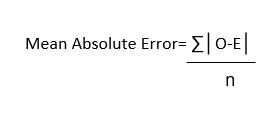Where,

• O stands for Observed values,
• E stands for Expected values,
• n stands for total no. of observations.

Now let us understand it with the help of an example.

Example:

Follow the below steps to calculate MAE in Excel:

Step 1: Suppose we have the following data: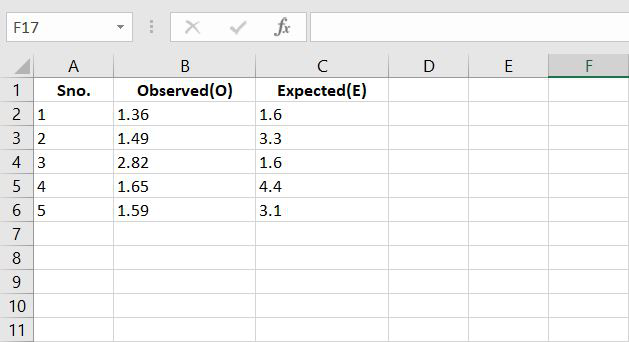Step 2: According to formulae, let’s calculate the difference between the observed and the expected values.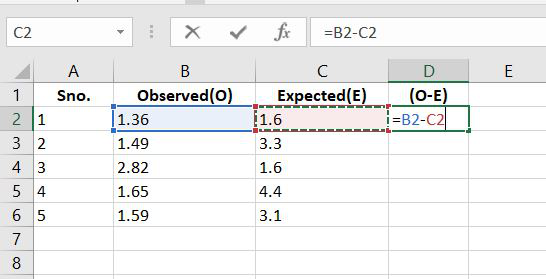Step 3: Now let’s calculate the absolute values.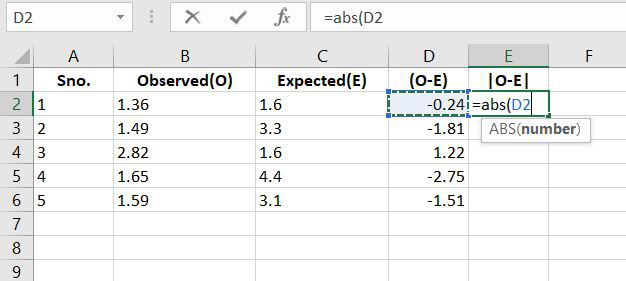Step 4: Now we know from the data that n= no of observations and in this the value of n=5. And also the let’s calculate the total sum of |O-E|.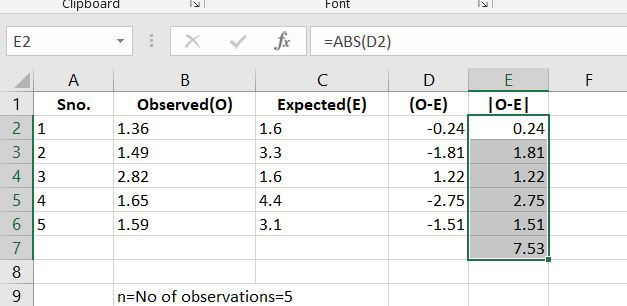Step 5: Now we have the value of Sum of |O-E| and the value of n. Now according to formulae, the Mean Absolute Error is calculated as: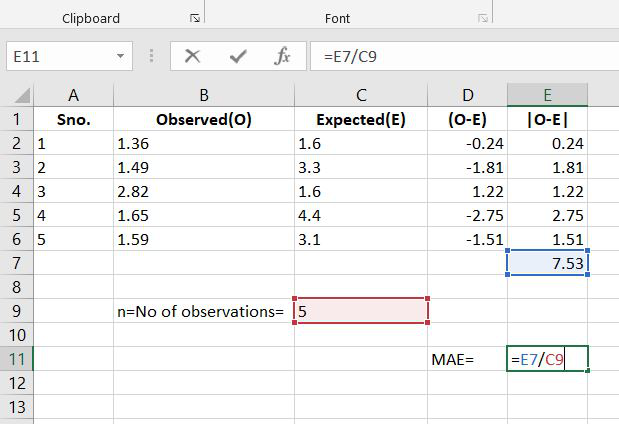Step 6: So the value of MAE is: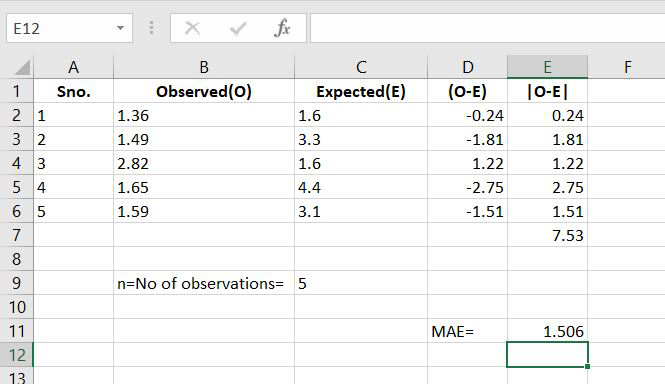Attention reader! Don’t stop learning now. If you are an Excel beginner (or an intermediate) and want to learn Excel, Geeksforgeeks brings the perfect course for you to start, Diving Into Excel

My Personal Notes arrow_drop_up